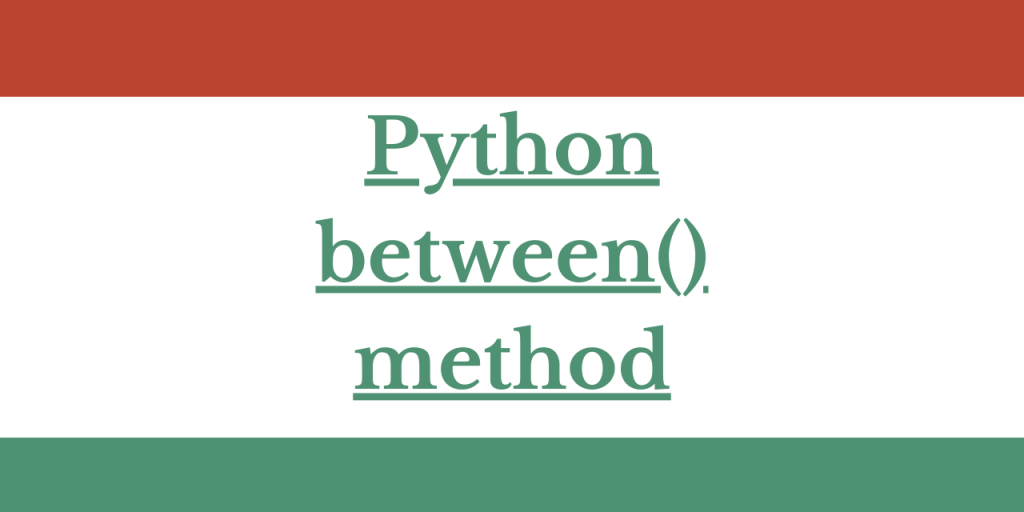# Python Pandas between(): Check If a Number is Between Two NumbersHello, readers! In our series of the Pandas module, we will discuss an unaddressed yet important function – Python Pandas between() function in detail.

So, let us get started!

The Python Pandas between() method offers a simplified analysis of data values residing in rows and columns, specifically for numeric types. By specifying a range (start and end), the function allows users to identify which data elements fall within this range. This is particularly useful for data comparison and pre-processing checks before further actions such as modeling.

## Use of the Pandas between() method

Python Pandas module is basically used to deal with the data value residing in rows and columns i.e. in a kind of table/matrix form. Within which, we often come across data variables holding values of numeric types.

Analysis and transformation of data are necessary before processing them to any kind of action such as modeling, etc.

In essence, the Python Pandas between() function streamlines data analysis by facilitating comparison operations and last-minute data verification. It’s a tool that not only checks whether a data value falls within a certain range, but also allows users to decide whether to include the boundary values in the range.

The between() function checks for the value present between the start and the end value passed to the function.

That is, amongst a range of values, it will check which data elements fall between the start and end value passed.

Let us now try understanding the structure of the same!

### Syntax of Pandas between() Method

Have a look at the below syntax!

```Series.between(start, end, inclusive=True)
```
• start: This is the starting value from which the check begins.
• end: The check halts at this value.
• inclusive: If True, it includes the passed ‘start’ as well as ‘end’ value which checking. If set to ‘False‘, it excludes the ‘start’ and the ‘end’ value while performing the check.

To add, Python Pandas between() function works well for numeric values and 1-dimensional DataFrames only.

Let us now try to analyze the function through some examples.

### 1. Python between() function with inclusive set to ‘True’

In this example, we have created a 1-D Dataframe using `pandas.DataFrame() `function.

Example:

```import pandas as pd
data = {"Roll-num": [10,20,30,40,50,60,70], "Age":[12,21,13,20,14,13,15], "NAME":['John','Camili','Rheana','Joseph','Amanti','Alexa','Siri']}

block = pd.DataFrame(data)
print("Original Data frame:\n")
print(block)
```

Output:

Have a look at the below dataframe!

```Original Data frame:

Roll-num  Age    NAME
0        10   12    John
1        20   21  Camili
2        30   13  Rheana
3        40   20  Joseph
4        50   14  Amanti
5        60   13   Alexa
6        70   15    Siri
```

Now, we have applied between() method on the ‘Age’ variable of the data frame.

With inclusive set to True, the between() function will consider all ages within and including 12 and 15. It then returns ‘True’ for rows where the ‘Age’ falls within this range.

```block["Age"].between(12, 15, inclusive = True)
```

Output:

As a result, it returns False for index 1 and 3 because, the values fall beyond the range 12 to 15.

```0     True
1    False
2     True
3    False
4     True
5     True
6     True
Name: Age, dtype: bool
```

### 2. How Does the Pandas between() Method React to Categorical Variables?

Now, let us see what it yields for a string or categorical data.

If we pass a string or non-numeric variable to the Pandas between() function, it compares the start and end values with the data passed and returns True if the data values match either of the start or end value.

Example:

```block["NAME"].between("John", "Joseph", inclusive = True)
```

Output:

As a result, only two values are returned to be True.

```0     True
1    False
2    False
3     True
4    False
5    False
6    False
Name: NAME, dtype: bool
```

### 3. Printing the values obtained from between() function

In this example, we will try to print the data that falls between 12 and 15 using Pandas between() function.

Example:

```btwn = block["Age"].between(12, 15, inclusive = False)
block[btwn]
```

Output:

As we have set inclusive to False, it will check for the values that lie between 12 and 15 excluding 12 and 15 itself. So, it outputs the rows with ‘Age’ as 13 and 14.

```     Roll-num	Age	NAME
2	30	13	Rheana
4	50	14	Amanti
5	60	13	Alexa
```

## Conclusion

And with that, we’ve demystified the pandas between() function! Any thoughts or questions? Don’t hesitate to leave a comment. Keep an eye out for more deep-dives into Python functions and until then, embrace the joy of learning!